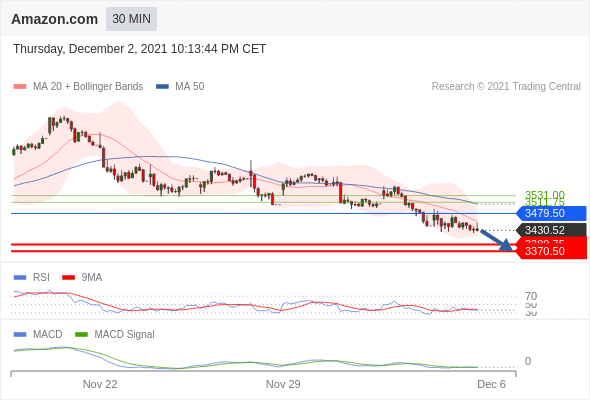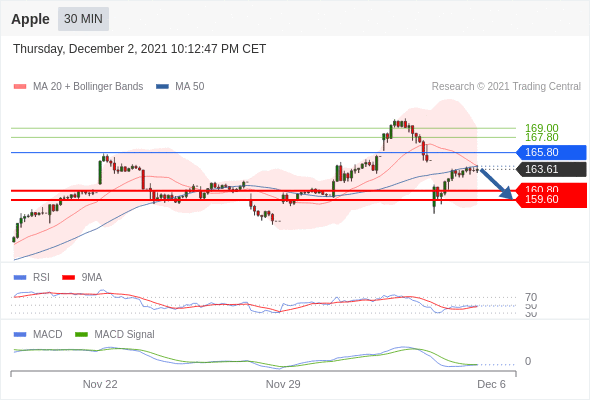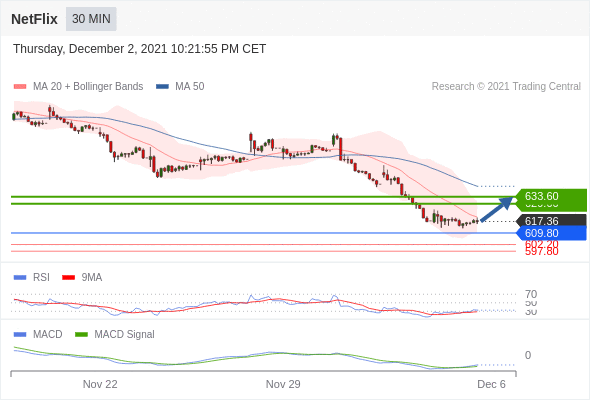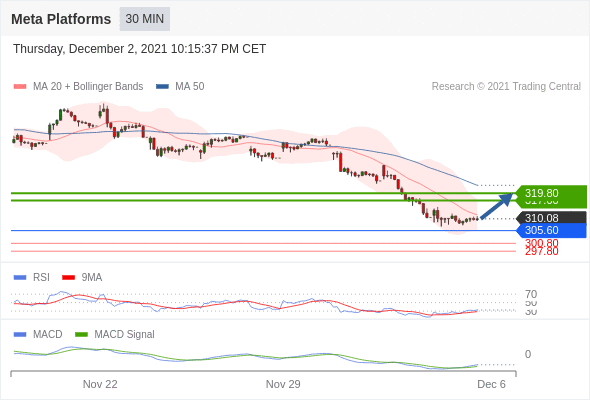top of page
Search

\$TSLA intraday: below its lower Bollinger band

Our pivot point is at 1114.4.

Preference: The downside prevails as long as 1114.4 is resistance.

Alternatively: The upside breakout of 1114.4, would call for 1139.09 and 1153.78.

Comment: RSI is below 50. MACD is negative and below its signal line. The configuration is negative. \$TSLA stands below its 20 and 50 period moving average (respectively at 1136.62 and 1128.57). Finally, \$TSLA is trading below its lower Bollinger band (standing at 1098.41).\$AMZN intraday: the downside prevails as long as 3479.5 is resistance

3479.5 is our pivot point.

Preference: The downside prevails as long as 3479.5 is resistance.

Alternatively: Above 3479.5, look for 3511.75 and 3531.

Comment: RSI is below 50. MACD is negative and below its signal line. The configuration is negative. \$AMZN stands below its 20 and 50 period moving average (respectively at 3457.11 and 3506.68).\$AAPL intraday: the downside prevails as long as 165.8 is resistance

165.8 is our pivot point

Preference: The downside prevails as long as 165.8 is resistance.

Alternatively: The upside breakout of 165.8, would call for 167.8 and 169.

Comment: The RSI is below its neutrality area at 50. The MACD is negative and below its signal line. The configuration is negative. \$AAPL stands below its 20 and 50 period moving average (respectively at 164.05 and 164.02).\$NFLX intraday: short term rebound towards 633.6

609.8 is our pivot point.

Preference: Short term rebound towards 633.6.

Alternatively: Below 609.8, expect 602.2 and 597.8.

Comment: RSI is below its neutrality area at 50. MACD is above its signal line and negative. The configuration is mixed. \$NFLX is trading under both its 20 and 50 period moving average (respectively at 620.29 and 640.55).\$FB (Meta Platforms) intraday: short term rebound towards 319.8

Our pivot point is at 305.6.

Preference: Short term rebound towards 319.8.

Alternatively: The downside breakout of 305.6 would call for 300.8 and 297.8.

Comment: The RSI is below 50. The MACD is negative and above its signal line. The configuration is mixed. Meta Platforms is trading under both its 20 and 50 period moving average (respectively at 311.69 and 322.8).\$MSFT intraday: the downside prevails as long as 333.7 is resistance

Our pivot point is at 333.7.

Preference: The downside prevails as long as 333.7 is resistance.

Alternatively: Above 333.7, look for 336.7 and 338.5.

Comment: RSI is below 50. MACD is negative and below its signal line. The configuration is negative. \$MSFT is trading under both its 20 and 50 period moving average (respectively at 331.42 and 333.26).